# Phase Lock Loop working and Phase detector, Loop Filter, and Voltage control Oscillator

(Last Updated On: September 11, 2021)

## Phase lock loop:

Starting from the computing to the communication the phase lock loops are widely used in many electronics applications. So in the communication they are used in the synchronization and the demodulation circuits. For example in the FM demodulation and in frequency shift keying they are commonly used. Apart from that when one wants to recover clock from the incoming bit stream then using the phase lock loop it is quite possible. Moreover in the communication system for jitter and noise reduction the phase look loop is commonly used. Then one of the most common applications of the Phase lock loop is the frequency synthesizers as well as the tone generation. So using the phase lock loop it is possible to generate which is multiple of the input frequency. In the microprocessor it is used in the generation of the stable system clock. So these are some of the common applications of the phase lock loops.

## Phase lock loop working:

So as its name suggests it is the control system or the control loop which maintains the same phase between the input and output signal. Now first we will understand that what the phase difference is?So in the above figure we have two signals which have same frequency but there is constant phase difference between the two signals.

While in the second figure we have the two wave forms start at the same time but they are of different frequency. So in this case the phase between the two signals is continuously changing with time.The phase lock loop synchronize the output signal with the input signal in the phase as well as in the frequency. So when the output frequency is equal to the input frequency and there is no phase difference or constant phase difference between the two signals then we can say that the loop is in the lock condition. The phase lock loop consists of 3 basics blocks which are:

• The phase detector
• Loop filter
• Voltage control oscillator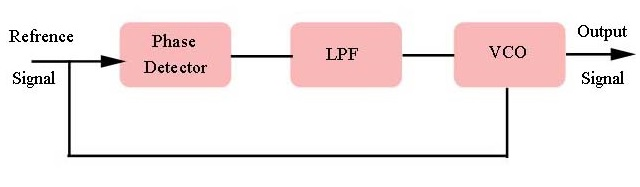In the voltage control oscillator when the voltage changes then the frequency of the oscillation also changes. So whenever the loop is just turned on then the VCO runs at the center frequency and this frequency is known as free running frequency. Now the phase detector comprises of the input or the reference signal with the oscillator frequency and based on that it generates the error signal. Now this error signal is passed through the low pass filter and the low pass filter generates the error voltage based on the error signal. On the basis of this error voltage the VCO either increase or reduce the oscillator frequency until the oscillator frequency locks to the input frequency. Under the lock condition there may be a no phase difference or the constant phase difference between the two signals.

## Capture range:

Now under the no lock condition the phase lock loop can acquire the lock only if the input signal is within the capture range of the phase lock loop. That means whenever the input signal is within the capture range then the VCO can lock to the input signal that means the capture range in the range of the input frequencies around the VCO centre frequency on to which the loop can lock whenever it starts from the unlock condition.

## Lock range:

Then the important specification of the Phase lock loop is lock range. So the lock range defines the range of the input frequencies over which the loop remains in the lock condition once it has captured the input frequency. So the VCO is already locked to the input frequency f. Now if the input frequency changes then the VCO will follow that frequency provided the input frequency is within the lock range. But if the input frequency goes out of the lock range then the VCO starts running at the free running frequency. It would not be able to lock to the input frequency until the input frequency is within the capture range of the phase lock loop. So there are the two important parameters of the phase lock loop.

## Phase detector:

So let us consider that at a given point of the time one signal has some phase ⍺ while another signal has some phase β that means the phase difference between the two signals is equal to ⍺ – β and if the phase difference is very small then we can say that sin⁡(⍺ – β ) is approximately equal to ⍺ – β and this sin⁡(⍺ – β ) can be written by the following expressions:

sin⁡(⍺ – β )=sin⁡⍺ cosβ- sin⁡β cos⁡⍺

From this expression we can say that when we multiply the two signals then we will get the two terms

sin⁡⍺ cosβ= sin⁡(⍺ – β )/2 + sin⁡(⍺+ β )/2

So the first term is the differences between the two phases while the second term is the summation of the two phases. So the second term is the high frequency signal and can be eliminated using the low pass filter that means just by multiplying the two signal it is possible to generate the phase difference between the two signals.
In the frequency domain if we see the same phenomenon then when we multiply the two signals then we get the two terms one is the summation of the two frequencies and the second is the difference between the two frequencies.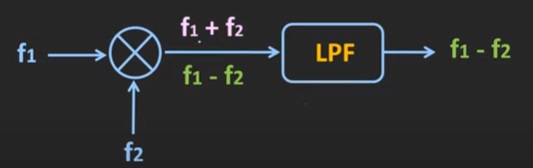So these high frequencies components can be eliminated using the low pass filter while the difference between the two frequencies contains the phase difference. When this signal is pass through the low pass filter and then it provides the required voltage for the VCO. So using this error voltage it is possible to lock the VCO at the input frequency. So add the radio frequencies the balanced mixer is used as a phase detector. While for the digital signal the phase frequency detector or even an XOR gate can be used for the phase detection. Now we will discuss how the XOR gate can be used a phase detector.

As we know that the output of the XOR gate will be high when the two inputs are different:

 X Y Output 0 0 0 0 1 1 1 0 1 1 1 0

Whenever the two signals are identical or whenever there is no phase difference between the two signals then the ideally output of the XOR gate should be equal to zero. But actually if we see we will find some spikes at the transitions. So if we take the average of these phase difference signal then it will be approximately equal to zero.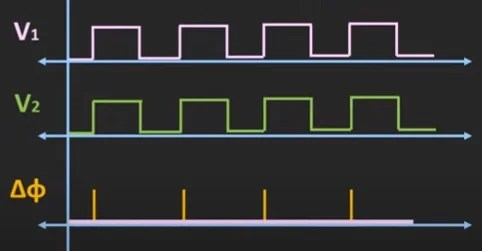Similarly when the phase difference between the two signals is 90 degrees then at the output we will get the waveform:When we take the average of this phase difference signal we will get the output voltage which is half of the peak value and whenever the phase difference between the two signals is 180 degrees then the output will be maximum and ideally it should be high for all time. But actually there will be some spikes at the transition. So if we take the average of this output waveform then it will be maximum value.

That means as the phase difference between the two signal increases, and then the average value of the output waveform will increase. So, this is the plot of the phase difference and the average output value of the low pass filter.So, as we can see, as the phase difference between the two signals increases from 0 to 180 degree, then the average output voltage will also increase linearly. That means this phase detector is the linear phase detector. But the phase relationship is linear only for 0 to 180 degree. Now, ideally, the phase detector should provide the linear phase relationship 0 to 360 degree. But in the XOR phase detector, as the phase difference increases beyond the 180 degree, then the average output voltage will start reducing. Moreover, it is difficult to identify, which signal is leading or lagging. For example, irrespective of V1 is leading or lagging the V2 by 90 degree, we get the same output waveform.

Apart from that, for the XOR gate to work as a linear phase detector, both signals should have approximately 50 percent of duty cycle and whenever the two signals are operating at the different frequencies then it is quite possible that the loop can get locked to the harmonic frequency. So, these are some of the problems with the XOR phase detector and these problems can be eliminated using the Phase Frequency Detector.

So, as shown in the figure, the phase-frequency detector is designed using the D-flip flop and the AND gate and the output of the detector is usually given to the charge pump circuit.So, here the output of the detectors is given to the PMOS and NMOS circuits. So, let’s quickly understand the working of this phase frequency detector. So, as we can see over here, the inputs of this both D-flip flops are connected to the logic high and the two signals between which we want to measure the phase difference are given as a clock signal to this D-flip flop.

 UP DOWN ACTION 1 0 Vout= VDD 0 1 Vout= 0 0 0 Vout= VDD/2 1 1 Clear

So, here the signals V1 and V2 are given as a clock signal to the D-flip flops. So, at the rising edge of these input signals, the output of the D-flip flop will become high and here these two flip-flops are marked as UP and DOWN. When the UP and DOWN outputs are high, then the output of the AND gate will also become high and that will RESET this D-flop flops. And once the D flip-flop receives the clear signal, then the UP and the DOWN outputs will become zero. So, in this particular case, when both UP and DOWN outputs are zero, then the output will get isolated from the detector circuit. In this case, the Vout is the output of this voltage divider circuit. So, in this particular case, it will be equal to Vdd/2. Now, whenever only this UP output is high, then the upper transistor will become high. Because of that, it will act as a short circuit and in that case, the Vout will become Vdd. Similarly, when the DOWN output is high, at that time, this lower transistor will become high and that will pull down the output Vout to the 0V. So, using this circuit is possible to identify which signal is leading or lagging. Moreover, if both signals are running at different frequencies then also it is possible to correct them.

For example here V1 is the input signal to the PLL and the V2 is the output of the VCO. So, as we can see, here V1 is running at a higher frequency. Moreover, this V1 signal is also leading the V2.So, at the rising edge of the V1 signal, the UP signal will become high and it will remain high, till the rising edge of this V2. Now, once again this UP output will become high at the rising edge of this V1 and it will remain high until the rising edge of this V2. So, in this case, as the V1 is leading the V2, so the UP output will become high. But on the other end, if V2 is leading the V1, or V1 is lagging the V2, in that case, this DOWN output will become high. So, from the UP and the DOWN output, it is easy to identify which input is leading and lagging. So, in this case, when the UP output is high, then the overall output voltage will be pulled up from the Vdd voltage to the Vdd/2. If we take the average of this output voltage, then it will be more than the Vdd/2. That means the VCO frequency will increase until it matches the input frequency.

Similarly when the input frequency is less than the oscillator frequencyin this case, as V2 is leading the V1, so, the down output will become high. And it will remain high, till the rising edge of this V1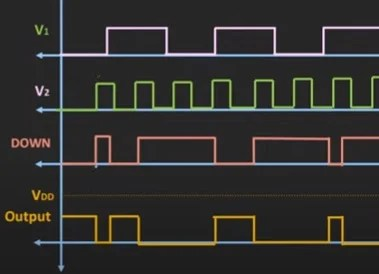Whenever, this down output is high, at that time, the overall output will be pulled down to the zero volt from the Vdd/2. If we take the average of the overall output waveform, then, in this case, it will be less than the Vdd/2. That means in this case, the control voltage of VCO will reduce. Due to that, it will bring down the VCO frequency. This is the basic working principle of this phase frequency detector. Now, as I mentioned earlier, the phase lock loop is commonly used in the frequency synthesizer circuit. So, in this basic PLL loop, when we introduce the divide by N counter in the feedback, then it is possible to multiply the output frequency by the factor of N.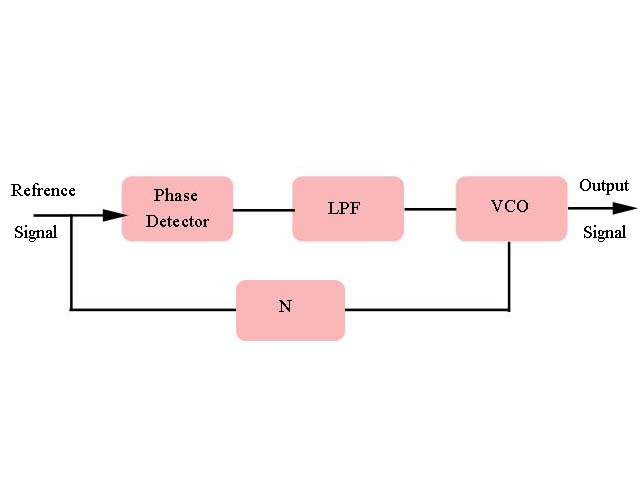The input frequency or the reference frequency to the PLL is equal to1 MHz and the VCO frequency is also 1 MHz when we introduce the divide by 10 counter in the feedback, then the output of that counter will be 100 kHz frequency which means the one input to the phase detector is 1 MHz frequency, while the second input is 100 kHz.

So, now the phase lock loop controls the VCO in such a way that, the output of the counter provides the 1 MHz frequency. That means the output of the VCO has to be at the 10 MHz frequency. Or in other words, we can say that the output of the phase lock loop is 10 times the input frequency. So, in this way, using this phase lock loop, it is possible to multiply the input frequency. Similarly, if we introduce this divide by N counter block before the phase detector, then it is possible to divide the output frequency by the factor of N which means that if fi is the input frequency, then the output frequency will be equal to fi / N. So, in this way, the phase lock loop can be used in the frequency synthesizers to multiply or divide the input frequency by the factor of N.